# Number theory derivation from infinity; speculations on equations that are derived in terms of the Field

## Recommended Posts

15 hours ago, studiot said:

I'm sorry, I have told you something very important about Fields and you have failed to address this point each time you have replied.
Instead you have introduced all sorts of irrelevant material.

I have failed to understand your point. I suppose I was working with a group of quadratic surds, and then looking at fields in terms of physics. Still doubt anything I derived is correctly done.

I'll leave off on further developments, and only refer to the work by Reg. Cahill for dynamical space and process physics as a better derivation alternative to GR than Suchard as a correction. That invalidates part of the premise, being that Cahill extends a flat space, but that seems like a fine way to wrap it up.

Edited by NTuft
*group
##### Share on other sites

• 2 weeks later...

On the issue of distant electric potential energy:

Universite' de Geneve`: De'partement de Physique Nucle'aire et Corpusculaire website: unige.ch
LHAASO Discovers a Dozen PeVatrons and Photons Exceeding 1 PeV and Launches Ultra-High-Energy Gamma Astronomy Era

Ultrahigh-energy photons up to 1.4 petaelectronvolts from 12 γ-ray Galactic sources

12 PeVatron energy level stars emitting from the region of Cygnus

web.archive link to English translation of Chinese Academy of Sciences article (May 24th, 2021):
LHAASO Discovers a Dozen PeVatrons and Photons Exceeding 1 PeV and Launches Ultra-high-energy Gamma Astronomy Era by Liu, Jia. May 17th, 2021

Quote

Although the accumulated data from the first 11 months of operation only allowed people to observe those sources, all of them emit so-called UHE photons, i.e., gamma rays above 0.1 PeV. The results show that the Milky Way is full of PeVatrons, while the largest accelerator on Earth (LHC at CERN) can only accelerate particles to 0.01 PeV. Scientists have already determined that cosmic ray accelerators in the Milky Way have an energy limit. Until now, the predicted limit was around 0.1 PeV, thus leading to a natural cut-off of the gamma-ray spectrum above that.

Zhen C., et al.

Cao, Z., Aharonian, F.A., An, Q. et al. Ultrahigh-energy photons up to 1.4 petaelectronvolts from 12 γ-ray Galactic sources. Nature 594, 33–36 (2021). https://doi.org/10.1038/s41586-021-03498-z

Nature abstract/info.:

Highest ever energy light captured by Chinese mountain observatory Science.org article by Ling Xin

Quote

Discovered more than 100 years ago, cosmic rays are charged particles, including protons and other atomic nuclei, that have been accelerated nearly to the speed of light. Their sources are poorly understood because interstellar magnetic fields bend them on their path to Earth. However, as cosmic rays rocket away from their sources, they also emit photons, usually about one-tenth as energetic as the cosmic rays themselves, that follow a straight path to Earth. Although Earth's atmosphere blocks this gamma ray light, when the photons slam into air molecules, they create showers of secondary particles and faint blue Cherenkov light that astronomers can look for.

Quote

LHAASO's core scientific goal is to explore the origin of high-energy cosmic rays and study related physics such as the evolution of the universe, the motion and interaction of high-energy astronomical celestials, and the nature of dark matter. LHAASO will extensively survey the universe (especially the Milky Way) for gamma ray sources. It will precisely measure their energy spectra over a broad range—from less than 1 TeV (trillion electron-volts or tera-electron-volts) to more than 1 PeV. It will also measure the components of diffused cosmic rays and their spectra at even higher energies, thus revealing the laws of the generation, acceleration and propagation of cosmic rays, as part of the exploration of new physics frontiers.

Quote

PEVATRONS AND PEV PHOTONS
The signal of UHE photons around PeVatrons is so weak that just one or two photons at PeV energy can be detected using 1 km2 of detectors per year even when focusing on the Crab Nebula, known as the “standard candle for gamma astronomy.” What's worse, those one or two photons are submerged in tens of thousands of ordinary cosmic rays. The 1,188 muon detectors in LHAASO's KM2A are designed to select photon-like signals, making LHAASO the most sensitive UHE gamma ray detector in the world. With its unprecedented sensitivity, in just 11 months, the half-sized KM2A detected one photon around 1 PeV from the Crab Nebula. In addition, KM2A found 12 similar sources in the Milky Way, all of which emit UHE photons and extend their spectra continuously into the vicinity of 1 PeV. Even more important, KM2A has detected a photon with energy of 1.4 PeV—the highest ever recorded. It is clear that LHAASO's scientific discoveries represent a milestone in identifying the origin of cosmic rays. To be specific, LHAASO's scientific breakthroughs fall into the following three areas:

1) Revealing the ubiquity of cosmic accelerators capable of accelerating particles to energies exceeding 1 PeV in the Milky Way. All the gamma ray sources that LHAASO has effectively observed radiate photons in the UHE range above 0.1 PeV, indicating that the energy of the parent particles radiating these gamma rays must exceed 1 PeV. As a matter of convention, these sources must have significances of photon signals five standard deviations greater than the surrounding background. The observed energy spectrum of these gamma rays has not truncated above 0.1 peV, demonstrating that there is no acceleration limit below PeV in the galactic accelerators.

This observation violates the prevailing theoretical model. According to current theory, cosmic rays with energies in the PeV range can produce gamma rays of 0.1 PeV by interacting with surrounding gases in the accelerating region. Detecting gamma rays with energies greater than 0.1 PeV is an important way to find and verify PeV cosmic ray sources. Since previous international mainstream detectors work below this energy level, the existence of PeV cosmic ray accelerators had not been solidly confirmed before. But now LHAASO has revealed a large number of PeV cosmic acceleration sources in the Milky Way, all of which are candidates for being UHE cosmic ray generators. This is a crucial step toward determining the origin of cosmic rays.

2) Beginning an era of “UHE gamma astronomy.” In 1989, an experimental group at the Whipple Observatory in Arizona successfully discovered the first object emitting gamma radiation above 0.1 TeV, marking the onset of the era of “very-high-energy” gamma astronomy. Over the next 30 years, more than 200 “very-high-energy” gamma ray sources were discovered. However, the first object emitting UHE gamma radiation was not detected until 2019. Surprisingly, by using a partly complete array for less than a year, LHAASO has already boosted the number of UHE gamma ray sources to 12.

With the completion of LHAASO and the continuous accumulation of data, we can anticipate to find an unexplored “UHE universe” full of surprising phenomena. It is well known that background radiation from the Big Bang is so pervasive it can absorb gamma rays with energies greater than 1 PeV. Even if gamma rays were produced beyond the Milky Way, we wouldn't be able to detect them. This makes LHAASO's observational window so special.

3) Photons with energies greater than 1 PeV were first detected from the Cygnus region and the Crab Nebula. The detection of PeV photons is a milestone in gamma astronomy. It fulfills the dream of the gamma astronomy community and has long been a strong driving force in the development of research instruments in the field. In fact, one of the main reasons for the explosion of gamma astronomy in the 1980s was the challenge of the PeV photon limit. The star-forming region in the direction of Cygnus is the brightest area in the northern territory of the Milky Way, with a large number of massive star clusters. Massive stars live only on the order of one million years, so the clusters contain enormous stars in the process of birth and death, with a complex strong shock environment. They are ideal “particle astrophysics laboratories,” i.e., places for accelerating cosmic rays.

The first PeV photons found by LHAASO were from the star-forming region of the constellation Cygnus, making this area the best candidate for exploring the origin of UHE cosmic rays. Therefore, much attention has turned to LHAASO and multi-wavelength observation of this region, which could offer a potential breakthrough in solving the “mystery of the century.”

Extensive observational studies of the Crab Nebula over the years have made the celestial body almost the only standard gamma ray source with a clear emission mechanism. Indeed, precise spectrum measurements across 22 orders of magnitude clearly reveal the signature of an electron accelerator. However, the UHE spectra measured by LHAASO, especially photons at PeV energy, seriously challenge this “standard model” of high-energy astrophysics and even the more fundamental theory of electron acceleration.

Off  1 order of magnitude:  0.1PeV estimate <  1.4PeV observed.

I also conjecture that the conclusion that the universe is nearly flat is preposterous if based on observations made from near here -- that until and unless we can position an observation position midway between ourselves and the purported edge the best we can say is that from our vantage it is "locally" flat, to get non-local we would have to measure from half way between or a sufficient vantage point.

Edited by NTuft
grammar
##### Share on other sites

You know, this is a really interesting game of

Who can make the most irrelevent post to the topic ?

Last night I was struck by an interesting observation in number theory.

What is the next number after 25 ?

Suprisingly the answer I came up with was 24 !

Any ideas how that can be ?

##### Share on other sites

On 7/17/2022 at 2:46 PM, studiot said:

You know, this is a really interesting game of

Who can make the most irrelevent post to the topic ?

Last night I was struck by an interesting observation in number theory.

What is the next number after 25 ?

Suprisingly the answer I came up with was 24 !

Any ideas how that can be ?

You were counting down???

On 6/30/2022 at 3:59 PM, swansont said:

How else do you get it to look like gravity, which has monopoles?

Are you familiar with Miles Mathis' work?

Quote

That oldest mistake is one that Euclid made. It concerns the definition of the point. Entire library shelves have been filled commenting on Euclid's definitions, but neither he nor anyone since has appeared to notice the gaping hole in that definition. Euclid declined to inform us whether his point was a real point or a diagrammed point. Most will say that it is a geometric point, and that a geometric point is either both real and diagrammed or it is neither. But all the arguments in that line have been philosophical misdirection. The problem that has to be solved mathematically concerns the dimensions created by the definition. That is, Euclid's hole is not a philosophical or metaphysical one, it is a mechanical and mathematical one. Geometry is mathematics, and mathematics concerns numbers. So the operational question is, can you assign a number to a point, and if you do, what mathematical outcome must there be to that assignment? I have exhaustively shown that you cannot assign a counting number to a real point. A real point is dimensionless; it therefore has no extension in any direction. You can apply an ordinal number to it, but you cannot assign a cardinal number to it. Since mathematics and physics concern cardinal or counting numbers, the point cannot enter their equations.

This is of fundamental contemporary importance, since it means that the point cannot enter calculus equations. It also cannot exit calculus equations. Meaning that you cannot find points as the solutions to any differential or integral problems. There is simply no such thing as a solution at an instant or a point, including a solution that claims to be a velocity, a time, a distance, or an acceleration. Whenever mathematics is applied to physics, the point is not a possible solution or a possible question or axiom. It is not part of the math.

Now, it is true that diagrammed points may be used in mathematics and physics. You can easily assign a number to a diagrammed point. Descartes gave us a very useful graph to use when diagramming them. But these diagrammed points are not physical points and cannot stand for physical points. A physical point has no dimensions, by definition. A diagrammed point must have at least one dimension. In a Cartesian graph, a diagrammed point has two dimensions: it has an x-dimension and a y-dimension. What people have not remembered is that if you enter a series of equations with a certain number of dimensions, you must exit that series of equations with the same number of dimensions. If you assign a variable to a parameter, then that variable must have at least one dimension. It must have at least one dimension because you intend to assign a number to it. That is what a variable is—a potential number. This means that all your variables and all your solutions must have at least one dimension at all times. If they didn't, you couldn't assign numbers to them.

This critical finding of mine has thousands of implications in physics, but I will mention only a couple. It has huge implications in Quantum Electro-Dynamics, since the entire problem of renormalization is caused by this hole in Euclid's definition. Because neither Descartes nor Newton nor Schrodinger nor Feynman saw this hole for what it was, QED has inherited the entire false foundation of the calculus. Many of the problems of QED, including all the problems of renormalization, come about from infinities and zeroes appearing in equations in strange ways. All these problems are caused by mis-defining variables. The variables in QED start acting strangely when they have one or more dimensions, but the scientists mistakenly assign them zero dimensions. In short, the scientists and mathematicians have insisted on inserting physical points into their equations, and these equations are rebelling. Mathematical equations of all kinds cannot absorb physical points. They can express intervals only. The calculus is at root a differential calculus, and zero is not a differential. The reason for all of this is not mystical or esoteric; it is simply the one I have stated above—you cannot assign a number to a point. It is logical and definitional.

This finding is not only useful in physics, it is useful to calculus itself, since it has allowed me to show that modern derivatives are often wrong. I have shown that the derivatives of ln(x) and 1/x are wrong, for instance. I have also shown that many problems are solved incorrectly with calculus, including very simple problems of acceleration.

This finding also intersects my first discoveries in special relativity, which I will discuss in greater detail below. The first mistake I uncovered in special relativity concerned Einstein's and Lorentz' early refusals to define their variables. They did not and would not say whether the time variable was an instant or a period. Was it t or Δt? Solving this simple problem was the key to unlocking the central algebraic errors in the math. Once it was clear that the time variable must be an interval or period, at least two of Einstein's first equations fell and could not be made to stand up again.

Next is my Unified Field Theory, just added to this list. I haven't put it above the correction to the point, since the correction to the point determined a part of my UFT. At the heart of my UFT is the discovery that Newton's gravitational equation is a compound equation, one that already includes the foundational E/M field or charge field. This pulling apart of Newton's equation by showing that G is a scaling constant between gravity and charge has become the new centerpiece of my website in the past few years, supplanting the number one spot held by my relativity papers. Since a NASA astrophysicist read my theory on this, recommended it, and wrote the introduction to my new book, this theory has generated a lot of interest worldwide. In it I also show that the current "messenger photon" cannot be virtual and that the field must be both real and mechanical. This means that Einstein's field equations are also compound equations. Einstein already had a UFT and didn't know it. But my theory goes far beyond this, since I don't just pull the lid off Newton and Einstein and then stand back. I segregate and simplify their equations, showing many many new things, including a correction to the perihelion of Mercury, a mechanical solution to the Metonic Cycle, and a new theory of tides. I also show that the universal gravitational constant G is a transform between the two constituent fields of Newton's equation. This allowed me to solve the dark matter problem, including the galactic rotation problem and the bullet cluster problem, by showing that the charge field outweighs normal matter by 19 to 1. Dark matter is not non-baryonic, it is photonic.

As the second part of this Unified Field Theory, I have also deconstructed Coulomb's equation. I show that Coulomb's constant k is tied to the Bohr diameter, and that when applied to quanta we can drop this constant from the equation. Like G, k is a scaling constant, and at the quantum level we have no need to scale. Among other things, this changes the force between electron and proton by a factor of 10-19. The charge part of this unified field has also allowed me to easily solve Bode's Law, resolving all the error, and to show the physical cause of axial tilt. Neither Bode's Law nor axial tilt are coincidences, as we have been told.

More recently I have found a third unified field equation: the Lagrangian. This was my most important discovery of 2010, and it must now rank very high in this list. I have shown that the kinetic energy variable in the Lagrangian is misassigned. Once the variable is properly understood in a fully mechanical two-part field, the Lagrangian becomes mathematically equivalent to my new Unified Field Equation, and I show in that paper how to go from one to the other in a couple of simple steps.

Most recently I discovered a fourth set of unified field equations, that being the equations of Maxwell. Specifically, I discovered that Maxwell's displacement field was hiding the charge field. This allowed me to tie many more things into my unified field, including Gauss' Law.

I haven't updated this paper in a long time, but my nuclear diagrams must certainly place high on this list. I am the first person in history to successfully diagram the nucleus, showing it is not just a bag of marbles. Rather, it is a complex channeler of charge. This has allowed me to also throw out electron bonding theory as unnecessary, as well as the strong force. I have now diagrammed most important elements and many important molecules, showing how their qualities are explained by these channels of charge.

http://milesmathis.com/central.html

Sure, lots of irrelevent material...

"Next is my Unified Field Theory, just added to this list. I haven't put it above the correction to the point, since the correction to the point determined a part of my UFT. At the heart of my UFT is the discovery that Newton's gravitational equation is a compound equation, one that already includes the foundational E/M field or charge field. This pulling apart of Newton's equation by showing that G is a scaling constant between gravity and charge has become the new centerpiece of my website in the past few years, supplanting the number one spot held by my relativity papers. Since a NASA astrophysicist read my theory on this, recommended it, and wrote the introduction to my new book, this theory has generated a lot of interest worldwide. In it I also show that the current "messenger photon" cannot be virtual and that the field must be both real and mechanical. This means that Einstein's field equations are also compound equations. Einstein already had a UFT and didn't know it. But my theory goes far beyond this, since I don't just pull the lid off Newton and Einstein and then stand back. I segregate and simplify their equations, showing many many new things, including a correction to the perihelion of Mercury, a mechanical solution to the Metonic Cycle, and a new theory of tides. I also show that the universal gravitational constant G is a transform between the two constituent fields of Newton's equation. This allowed me to solve the dark matter problem, including the galactic rotation problem and the bullet cluster problem, by showing that the charge field outweighs normal matter by 19 to 1. Dark matter is not non-baryonic, it is photonic.

As the second part of this Unified Field Theory, I have also deconstructed Coulomb's equation. I show that Coulomb's constant k is tied to the Bohr diameter, and that when applied to quanta we can drop this constant from the equation. Like G, k is a scaling constant, and at the quantum level we have no need to scale. Among other things, this changes the force between electron and proton by a factor of 10-19. The charge part of this unified field has also allowed me to easily solve Bode's Law, resolving all the error, and to show the physical cause of axial tilt. Neither Bode's Law nor axial tilt are coincidences, as we have been told.

More recently I have found a third unified field equation: the Lagrangian. This was my most important discovery of 2010, and it must now rank very high in this list. I have shown that the kinetic energy variable in the Lagrangian is misassigned. Once the variable is properly understood in a fully mechanical two-part field, the Lagrangian becomes mathematically equivalent to my new Unified Field Equation, and I show in that paper how to go from one to the other in a couple of simple steps.

Most recently I discovered a fourth set of unified field equations, that being the equations of Maxwell. Specifically, I discovered that Maxwell's displacement field was hiding the charge field. This allowed me to tie many more things into my unified field, including Gauss' Law." ...

This is also regarding your mention of, "it reduces to Newtonian gravity", which I asked for clarification about because I don't understand. I have not heard any new reports from MoEDAL experiment which I think is also up and running.

##### Share on other sites

1 hour ago, NTuft said:

Are you familiar with Miles Mathis' work?

Please don’t play games. If you have relevant information, post it.

##### Share on other sites

On 6/18/2022 at 7:57 AM, exchemist said:

I’m afraid it looks very much as if your hovercraft is full of eels.I think this thread's gone to meet its maker. It's joined the choir invisible. If it wasn't nailed to the forums topics it would be pushing up the daisies.------------

Honestly. I see no promising science here.

My summary: No. Monopoles are not dipoles for very much the same reason why monocles are not binoculars: two is not one.

Gravity and electromagnetism are not the same.

Radiation fields decrease with distance as (distance)-1; monopolar fields decrease with distance as (distance)-2; dipolar fields fall off as (distance)-3. That's another reason why they can be told apart. Beside the ones explained by myself and others. Each one varies with distance as the spatial gradient of the previous-order one.

Plus EM doesn't satisfy the equivalence principle. Only repetition, and familiarity with it (the EP) makes you forget how incredibly surprising it is, how much different the world would be if EM and gravity were more like "different versions of the same thing," and how exceptional gravity is. The 1/r2 coincidence is but a mirage.

Plus EM is scale-independent while gravity is scale-dependent.

Plus charge is a relativistic invariant, while mass is not.

Plus...

And still I can't see what any of this has to do with number theory, which AFAIK has no relevance to physics,* as the question whether, say, the speed of light, or the mass of a body, or any other physical quantity is a rational or irrational number (or prime, or has any other number-recursion connection) doesn't really make sense, for the very simple reason that any of those are measured numbers, and inevitably go with a range of experimental precision. So any claim that you would want to make in terms of number theory would immediately be whitewashed by the fact that all physical quantities are cut off by virtue of experimental uncertainties.

On 6/25/2022 at 3:13 PM, studiot said:

Especially relevant.  +1

I stopped bothering with this thread since it left the title subject of number theory far behind.

My feelings exactly.

* Repeat: Number theory has no relevance to physics as both stand today. If it has, IMO, you should make a strong case why it does, if you think it does. There's nothing written in stone that says that number theory and physics will never be related in the future.

##### Share on other sites

On 7/20/2022 at 2:56 PM, swansont said:

Please don’t play games. If you have relevant information, post it.

Here is the complex graph of y=+(1/x):

Graphing Q1 (+/+) and Q3 (-/-) Cartesian quadrants. Not graphed Q2(+/-) and Q4 (-/+): y=-(1/x). So this complex plane contains a spherical discontinuity at the origin. If we take all square roots of prime numbers to be variables for complex math I think the discontinuity becomes an ellipsoid and the hyperbolas upon rotation are providing conics. I think extensions of complex numbers start running into problems, and what can be done with quaternions, octonions, or sedenions as setting up iterations with more and more "imaginary" components points towards imaginary numbers having a natural extensibility. I think real variables are appended to the i value to create any multiples needed, but it is better math to have each i with a possible set of values. This may create limitations, or the real number multiplier may still be valid, but something would have to change with the exponentiation rules for equating or simplifying in. Perhaps the i,j,k, or , or  are like polynomials, and since if there were different primes assigned to different values there'd be constraint on how the complexity could be reduced, which could be used to assign quantum states to influence other aspects of states as versors: electron spin state influences magnetic spin state, and azimuthal number and principal number are related; so measuring one collapses some possibilities and the measurement has some inherent uncertainty which I think we need to use to account for the discrepancy in the virial theorem for multi-atom systems.

On 7/20/2022 at 3:58 PM, joigus said:

[...]

Honestly. I see no promising science here.

My summary: No. Monopoles are not dipoles for very much the same reason why monocles are not binoculars: two is not one.

Gravity and electromagnetism are not the same.

Radiation fields decrease with distance as (distance)-1; monopolar fields decrease with distance as (distance)-2; dipolar fields fall off as (distance)-3. That's another reason why they can be told apart. Beside the ones explained by myself and others. Each one varies with distance as the spatial gradient of the previous-order one.

Plus EM doesn't satisfy the equivalence principle. Only repetition, and familiarity with it (the EP) makes you forget how incredibly surprising it is, how much different the world would be if EM and gravity were more like "different versions of the same thing," and how exceptional gravity is. The 1/r2 coincidence is but a mirage.

Plus EM is scale-independent while gravity is scale-dependent.

Plus charge is a relativistic invariant, while mass is not.

Plus...

And still I can't see what any of this has to do with number theory, which AFAIK has no relevance to physics,* as the question whether, say, the speed of light, or the mass of a body, or any other physical quantity is a rational or irrational number (or prime, or has any other number-recursion connection) doesn't really make sense, for the very simple reason that any of those are measured numbers, and inevitably go with a range of experimental precision. So any claim that you would want to make in terms of number theory would immediately be whitewashed by the fact that all physical quantities are cut off by virtue of experimental uncertainties.

[...]

* Repeat: Number theory has no relevance to physics as both stand today. If it has, IMO, you should make a strong case why it does, if you think it does. There's nothing written in stone that says that number theory and physics will never be related in the future.

From number theory proposition, we will use versors to describe quantum objects. There's the primary quantum number, electron spin quantum number, induced magnetic quantum number, and azimuthal quantum number. I think primary and aziumuthal quantum number are tied together but I need to review. Electron spin state induces magnetic spin state, but magnetic spin state can have complexity that may be playing into uncertainty.

An aside on the, uh... dipole... we have a brick of metal. If in this brick of metal the electron spin states align pointing the same way, given a... gradient... to measure against, it is magnetized. The magnetic dipole: it is induced by current at the quantum level from electrons, and is consisted of a condensed brick of iron, and, nowadays, neodymium dysprosium or something else and boron-doped bricks. If it's in motion, there may be Magnetohydrodynamics involved and I don't get it yet.

With a complex number describing 1 real time dimension and three complex spatial dimensions we describe the transformations that are possible. Measurement of spin number tells of magnetic quantum number. Measurement of primary quantum number tells of azimuthal number. Compression/torsion as described in higher-dimensional algebraic geometry structures encodes potential energy discrepancy and I think needs to be written into as describing a physical field; I think physics here is of course dependent on number theory directly through complex numbers as objects for creating these spaces.

Another aside, I'd say the solutions to Riemann's zeta function and his hypothesis being correct follows from it being literally a geometric series exponentiating (limited) complex numbers in the denominators, and this creates a logarithmically spiraling sinusoid if graphed vertically, and this is tied to Fourier transformation and harmonic oscillators. I think if complex numbers were expanded to include all square roots of primes the trivial zeros at negative even integers and the pole at +1 would change in the zeta function, but I don't know that, and this is perhaps the primary number theory speculation here. The Riemann Zeta function is able to bound prime number distribution with a low error rate because it is creating an algebraic geometric construction to graph them as bounded by a logarithmically distending, vertical sinusoid wave: graphed vertically the +(1/2) real part, "non-trivial" zeros form a vertical pole of point hits just under the bound of the real pole at +1. This is the most accurate point for the "hits" to occur, hence the low error rate. When the sinusoidal wave is traveling in the harmonic oscillator models we are using the complex unit circle trigonometric relations, and so Fourier transformations translate vibrational data by extending the complex unit trigonometric functions but I think they're inaccurate because they need to based on hyperbolic geometry and a wider set of imaginary numbers, which are related by right-angle trigonometry.

A concept in number theory can be obtained from taking a specific number set under an operation, conceptualized as being derived from 5-D where, perhaps charge seperation effects are equivalent to motion, as in Kaluza-Klein theory which I just read about. However, I think time is simple and limited to one-dimension, and space is complex and needs the more representative part of complex numbers, like with quaternions there could be 3 complex spatial dimensions and one real time dimension. Then, literally reducing dimensionality of the field through the differentiation operation of  to 4-D where the simple time dimension is incorporated, to where making any measurements have an uncertainty to account for the virial deviation for even simple molecules: it should be set at +/- 40% given that the virial theorem cannot account for a simple molecule by a 20% discrepancy (2.0 vs. ~1.61). I conjecture that the complex hyperbolic graph is cutting cones, akin to light cones of radiant light events in spacetime, and that the ellipsoid discountinuity is equatable to an uncertainty in the time-measurement of the system: the displacement out along the x or y-axes (with the x-axis discontinuity narrower and y-axis discontinuity containing the focii of the ellipse once on an exponentiated hyperbolic plane) covers a length which is tied to the uncertainty in the measurement. Also this radiance outwards should be differentiated from emanations, which I envision akin to stored torsion or compression that'd have helical or differently spiraled propagation: and again I think cutting conics from the hyperbolas can be descriptive.

Fourier transformations which seem inaccurate by being unable to account for uncertainty in vibrational spectra, but, that is just my opinion. I don't know yet how to do the actual math required to check if this works, or to improve the accuracy in spherical/elliptical/hyperbolic harmonic functions which should function as descriptors of molecular orbitals and perhaps also pair or groups of nucleons. But, the juiced up compression/torsion forces encoded by quaternions or further complexifications of specific algebras can perhaps better describe complex quantum systems by having exponentiations in the quantum numbers. Even the primary quantum number for simple absorbance spectra should be scaled, as normal integers do not describe the change in vibrational rate; groups of square roots of primes could be made to be degenerate energy states if necessary for principal quantum number and can better describe the other 3 quantum numbers and their implications upon measurement: a potential energy shift encoded by switching spin states, thereby magnetic spin states, or azimuthal position as encoded by measurement uncertainty with regards to time as to whether the quantum object was at an actual discrete position or undergoes a quantum tunneling wave probabilistic transition to another allowed state (e.g. +1/2 vs. -1/2 spin state, it's implications on magnetic spin state; or principal number 1-6+ excitation, and it's implication on azimuthal number occupancy) which could be like going back in time, or more like the measurement gives a result from a prior part of the interval, akin to antimatter generation speculations. To account for the -20% incorrect measure in virial (2.0 vs. 1.61) we increase the bound to +/-40% potential energy under a rubric utilizing complex hyperbolic or conic harmonic oscillators, and I think this has implications for the physics of accounting for charge/mass/energy.

This speaks to charge potential that is not accounted for properly in the current paradigm. Current accounting alludes to dark matter, dark energy, and also the existence of magnetic monopoles (as yet unseen) as a proof of the quantization of charge. Therefore I think there is insufficient reason to conclude that charge is quantized, but I do not understand that quantization, or what the implications are for total charge density. However, as an explanation, I will try to learn what @joigus is talking about by -1-2, and -3 decrases in force vis visa gravity and electromagnetism, and to understand the equating of inertial masses with gravitational masses--the equivalence principle! I want to equate mass and charge, and claim that charge is not properly being measured, perhaps as a result of being unable to account for electric field lines actually having no closure and entering singularities like White or blackholes (Q1 and Q3 and Q2 and Q4 for complete quadratics?). So this is also about the issue of monopoles, and if they were supposedly plentiful earlier in the evolution of the universe, or where they've gone, too. I did not equate monopoles to dipoles, I've only just now tried to define a dipole and that probably needs correction.

On physics and math here broadly,

Quote

The four-dimensional algebra of hyperbolic quaternions incorporates some of the features of the older and larger algebra of biquaternions. They both contain subalgebras isomorphic to the split-complex number plane. Furthermore, just as the quaternion algebra H can be viewed as a union of complex planes, so the hyperbolic quaternion algebra is a union of split-complex number planes sharing the same real line.

It was Alexander Macfarlane who promoted this concept in the 1890s as his Algebra of Physics, first through the American Association for the Advancement of Science in 1891, then through his 1894 book of five Papers in Space Analysis, and in a series of lectures at Lehigh University in 1900.

speaks to some precedent in relevance between physics and number theory, which number theory I think includes complex math as mathematical objects for creating things like Lie algebras, or groups of algebraic expressions that are being used to describe physical fields.

If anyone can talk me out of my mathematical misadventure regarding imaginary numbers, I'd appreciate it. Can the rules of exponentiation that are used to simplify be re-written? As even exponents given multiples (+1 or -1) and odds give multiplies times roots (+1+i or -1-i)? Or does this create inoperability of quaternions or octonions as their division, multiplication, addition or subtraction is complexified? Doubt it makes sense. Do please speak to this vis viva number theory: can we take the set of square roots of primes as the object in complex numbers ()? Might this put legitimacy to complex math as based on the harder to define but equally consistent two products of quadratics +/- and -/+ as opposed to +/+ and -/- which we have short-hand for in normal polynomial roots? Wouldn't expanding the definition of complex numbers in this way effect Fourier transformations in particular, which touches the gradients of fields, expansion of harmonic functions, etc.?

I know there are problems with the Exponentiation: Failure of power and logarithm identities.
I want to re-iterate that the prime number distribution π(X) ∼ X log X ⇐⇒ ψ(X) ∼ X and the Zeta function creating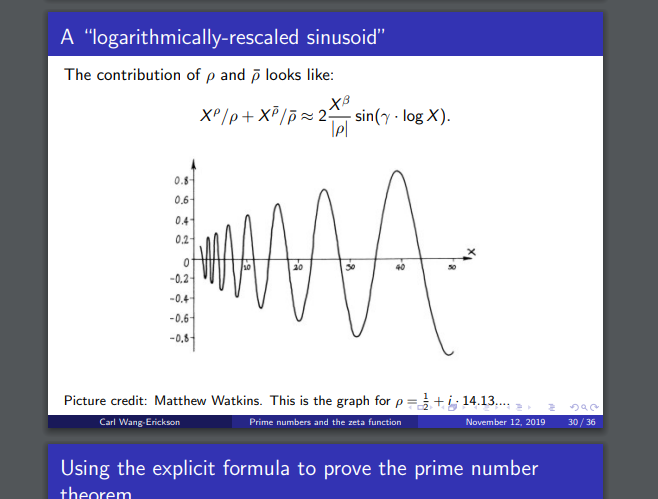as seen here  Prime numbers and the Riemann zeta function, Undergraduate Mathematics Seminar, University of Pittsburgh, November 12, 2019. 36 slide presentation, by Carl Wang-Erickson may be due to the availability of all prime numbers to be objects for complex math, and that this is

Quote

Number theorists study prime numbers as well as the properties of mathematical objects made out of integers (for example, rational numbers) or defined as generalizations of the integers (for example, algebraic integers).
[...]
Starting early in the nineteenth century, the following developments gradually took place:

• The rise to self-consciousness of number theory (or higher arithmetic) as a field of study.
• The development of much of modern mathematics necessary for basic modern number theory: complex analysis, group theory, Galois theory—accompanied by greater rigor in analysis and abstraction in algebra.
• The rough subdivision of number theory into its modern subfields—in particular, analytic and algebraic number theory.

is about number theory, and if it is to change imaginary numbers that has direct implications for physics regarding harmonic oscillators, Fourier transformations, and other aspects of what I'd formerly described as quantum chemistry. I think this works towards two other Millennium prizes, but, I can't actually do the math.

"Plus charge is a relativistic invariant, while mass is not." Ought verify understanding of that, EP, -1,-2,-3, more basic math, EM and mono-/di-pole magnets, actual details of quantum numbers, other speculations.

Thanks

##### Share on other sites

On 7/28/2022 at 11:41 PM, NTuft said:

From number theory proposition, we will use versors to describe quantum objects.

What do you mean versors? And what properties of those entities describe interference, polarization, helicity, tunneling, spin-statistics connection, or ground-state energy? All of them known and measured properties of quantum objects.

If you actually described any one of those properties from number theory, I'd take your ideas very seriously.

##### Share on other sites

In response to the last superlarge dish of word salad I offer a simple analysis, I offered on a maths site to someone who thinks they have discovered a 'pattern' in the occurrence of primes.

Since there are a very large number of primes (the ancient Greeks proved this large number to be transfinite) it is not only not suprising to be able to find not only a pattern but any finite pattern inclusded in that infinity.

##### Share on other sites

On 7/29/2022 at 10:26 PM, studiot said:

In response to the last superlarge dish of word salad I offer a simple analysis, I offered on a maths site to someone who thinks they have discovered a 'pattern' in the occurrence of primes.

Since there are a very large number of primes (the ancient Greeks proved this large number to be transfinite) it is not only not suprising to be able to find not only a pattern but any finite pattern inclusded in that infinity.

Yes well I've not figured how to route the obstructionism about number theory's definition and modern developments you're providing succinctly.

How about finite fields have a prime for the modulus? The p-adics systems as equally workable as the reals? How about the derived equation I posited is a function acting on a set of integers?

Since ancient times we've gone on to complex analysis, unless you're some math luddite or reductionist in your rigor.

On 7/29/2022 at 9:55 PM, joigus said:

What do you mean versors? And what properties of those entities describe interference, polarization, helicity, tunneling, spin-statistics connection, or ground-state energy? All of them known and measured properties of quantum objects.

If you actually described any one of those properties from number theory, I'd take your ideas very seriously.

Versors as I understand are quaternions describing vectors. Using a prime number system that is restricted under operation I think can describe physical properties, ad hoc, but would that interfere with what are supposed to be translations in the vector field? A prime number based system should be able to assign values to variables as well as the real numbers, and I think quantization, irreducibility, or rules for interactions could be indicated by a prime root.

##### Share on other sites

7 hours ago, NTuft said:

Using a prime number system that is restricted under operation I think can describe physical properties, ad hoc,

How?

OTOH, I'm sure you know ad hoc explanation, or "just so" stories, are not good enough in science. They're lacking in the falsifiability aspect.

##### Share on other sites

• 4 weeks later...
On 7/31/2022 at 3:12 AM, joigus said:

How?

OTOH, I'm sure you know ad hoc explanation, or "just so" stories, are not good enough in science. They're lacking in the falsifiability aspect.

The only aspect I had a grasp of for that was quantum number interdependences, but no, I'm not sure that's applicable or how quaternions and octonions are used in describing physical systems. From rereading the Quanta Magazine piece about Furey ("The Strange Math..."),

Quote

“I realized that the eight degrees of freedom of the octonions could correspond to one generation of particles: one neutrino, one electron, three up quarks and three down quarks,”

I was thinking of the quaternions to describe the four quantum numbers (i.e. an electron at my QM level), but that would imply that the math operations (are they just changing directions?) correspond to the physical relations and I think that is incorrect. However, being that quaternions are used in computer graphic modeling of 3-D rotations an ad hoc description system could have each atom or quantum object as a sphere that is then acted upon by other bodies in the system, say for modeling collisions or bond formation. I need to read it, but there is the precedent of bi-quaternions being formulated into physics as previously mentioned and again from the QM article there is the octonion numbers being used for physical systems. Last and not least, if instead of being underpinned by the real numbers, the real foundation is the square roots of primes, the 4 normed division algebras and possibly the continued dyadics sedenions trigintaduonions... are the real foundation and are more easily operable than real numbers, if I understood anything about p-adics. I doubt there even needs to be a real number appended to the front of the quaternion or octonion group, which could remove some of the difficulty of real numbers compared to p-adics. It looks as though the real number is the resultant of an i'2 or additives thereof that have removed the root operation, whereas the i,j,k or e1-ehave a different prime under operation that creates an incompatibility under multiplication or division and hence the noncommutativity and/or nonassociativity, but, this is just more speculation; I don't know if there is anything here falsifiable or testable for you.

More complex physical system descriptors I think you'd know better about, although I mentioned compression... but allow me to move to a presentation on some information on the, "...quirks of the quaternions being noncommutative and octonions even nonassociative, they continue to find uses in fields such as algebra, geometry, topology and number theory"

Thanks

Edited by NTuft
grammar; scattershot mistake; undefined terms.
##### Share on other sites

33 minutes ago, NTuft said:

I was thinking of the quaternions to describe the four quantum numbers (i.e. an electron at my QM level), but that would imply that the math operations (are they just changing directions?) correspond to the physical relations and I think that is incorrect.

As it stands today, quaternions are used as an alternative representation of the Dirac equation. The 4 components would correspond to the 4 components of the Dirac spinor, which are interpreted as both spin states of electron and positron. I'm not aware of any full-fledged formulation of QED based on quaternions. I don't know much more about this idea.

As to octonions... mmm. I don't know. They are non-associative, which is, right off the bat, quite strange to represent physics. But who knows.

##### Share on other sites

6 minutes ago, joigus said:

As to octonions... mmm. I don't know. They are non-associative, which is, right off the bat, quite strange to represent physics. But who knows.

To pick a bone, fwiw,
"[Octonions] are noncommutative and nonassociative, but satisfy a weaker form of associativity; namely, they are alternative. They are also power associative."
Octonion handwiki.org

"In abstract algebra, an alternative algebra is an algebra in which multiplication need not be associative, only alternative. That is, one must have

for all x and y in the algebra.

Every associative algebra is obviously alternative, but so too are some strictly non-associative algebras such as the octonions."
Alternative algebra handwiki.org
"An algebra (or more generally a magma) is said to be power-associative if the subalgebra generated by any element is associative. Concretely, this means that if an element  is performed an operation * by itself several times, it doesn't matter in which order the operations are carried out, so for instance
"

##### Share on other sites

41 minutes ago, NTuft said:

To pick a bone, fwiw,
"[Octonions] are noncommutative and nonassociative, but satisfy a weaker form of associativity; namely, they are alternative. They are also power associative."
Octonion handwiki.org

"In abstract algebra, an alternative algebra is an algebra in which multiplication need not be associative, only alternative. That is, one must have

for all x and y in the algebra.

Every associative algebra is obviously alternative, but so too are some strictly non-associative algebras such as the octonions."
Alternative algebra handwiki.org
"An algebra (or more generally a magma) is said to be power-associative if the subalgebra generated by any element is associative. Concretely, this means that if an element  is performed an operation * by itself several times, it doesn't matter in which order the operations are carried out, so for instance
"

Do you understand what this is all about and can you relate it to the thread topic of prime numbers and Fields ?

##### Share on other sites

5 hours ago, studiot said:

Do you understand what this is all about and can you relate it to the thread topic of prime numbers and Fields ?

As I understand it, if each bit of a complex number had a different root prime, operations would be under certain rules. So, as an example, if
and
, then

.

I am unsure if within a quaternion the root primes should be the same, but I think there's an irreducibility about it that could explain the way translations (i.e. changes in direction) are described. The identity element 1 may be necessary, so NO, I do not understand what this is all about.

##### Share on other sites

• 2 weeks later...

I'll try to summarize what comes out to me with LaTeX pasted into here. I think the multiplication of the basis elements is going to change from being unital because I'm going to change the definition of the imaginary unit, so and squares come out here and in the periodic/cyclic exponentiations:

if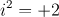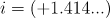or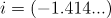or

soor;orTo try to maintain the aspect of periodicity or cyclic exponentiation from the established basis, {1, i, j, k), I look to cycle the signs for exponentiation here as:
+i, -1, -i, +1
+, -, -, + ; orso, +, +, -, -
HOWEVER,, so trying to flip the signs accordingly given that we're using a positive prime:
so +, +, -, -, when using the established imaginary unit-, -, +, +,
since the attempt is now to equate a positive prime number as imaginarybut, this is problematic, as it looks like my algebra is falling apart. I'm not too surprised given that I'm trying to equate, which is unconventional; but, there is the aspect thatcould be showing here. I don't know about how exponentiation of i can hold; this may well break the idea's viability. What do you think about how this would affect geometry? To me, this is now scaling what was formerly a unit circle, and it is now perhaps an exponentiated shape? A scaled hyperbolic complex graph? Please try to think about it and write back your thoughts or ideas.? What I am equating here is the equivalency of roots I'd mentioned. ++, -- = +-, -+? I think this may be related to +i, -i convention, but, again, this is problematic. See attachment for how I try to use this ideanegative prime, so +, +, -, -; exponentiation cycle:These are examples for a positive and a negative prime. Please write back and let me know what you think. I think there are problems. I have gone too far -- I will claim +1s and -1s are primes, I will saythen put in garbage likeand pull a switch-er-oo to havefollow it. This must offend you so deeply that you will be compelled to respond, having fashioned a dunce cap and able to explain the Lies in my algebra. Or you must lament my wanton signifying.

Edited by NTuft
##### Share on other sites

• 2 weeks later...

LaTeX from last post: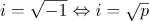iforor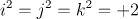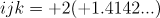or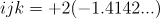or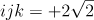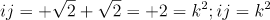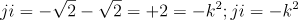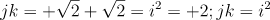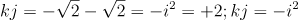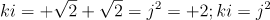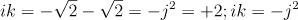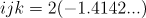or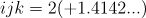for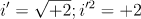, the signs coming from exponentiation flip accordingly given that we're using a positive prime:
+i, -1, -i, +1
+, -, -, +
or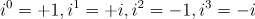so +, +, -, -, when using the established imaginary unit
so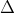to  -, -, +, +, to equate a positive prime number as imaginary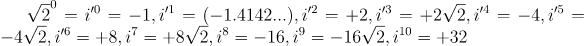if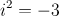or  or  or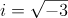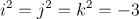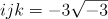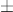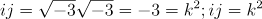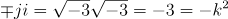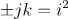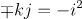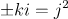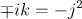exponentiation cycle, signing similar toso +, +, -, - :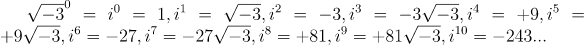Please critique or make input. Question whether one quaternion could be of form:
with , and  and if this is useful. No speculation on octonions as I don't understand quaternions.

***PLEASE IGNORE BELOW I NEED TO FIGURE OUT PROPER SITE LATEX***
Edited by NTuft
disclaimer re:annoyances
##### Share on other sites

• 1 month later...

Staggered geometric progressions or logarithmic spirals that are created on the Argand plane by expanding definition of imaginary numbers because of the exponentiations. Even numbered exponentiations generate real numbers and odd numbered generate imaginary since working with purely imaginary numbers, so points on the graph are mapped to the axes. Solid line and numbering for +i and -real axes for graphical description given at upper rt.-hand corner describing CCW rotation(from +i axis for positive prime); dashed line and numbering for -i and +real axes for representation described in upper lt.-hand corner describing CW rotation (from -i axis for negative prime). Note that increments for  on imaginary axis go by multiples of  and by +1's and -1's on the real axis, and for  the increments are in 10's of  and blocks of 10 on the real axis; as denoted by solid and dashed lines, respectively.

Uncertain how to properly scale axes, but I do think there is a geometric progression because each time there is a squared exponentiation there is a multiplication here by 2 or 7, or by each raised power a multiplication by the sqaure root of those; also unknown if negative exponentiation should instead create a descreasing spiral or the symmetry (change in rotation direction?) around zero as described previously: confusion d/t complex numbers since negative exponents should mean how many times to divide by the number but on the complex plane exponentiation is creating rotation...

Edited by NTuft
grammar, clarity.
##### Share on other sites

• 2 months later...

@studiot, @joigus, @swansont, @uncool, @Genady, @dimreepr, @StringJunky, @Markus Hanke, @Mordred, et al.,
https://www.claymath.org/sites/default/files/yangmills.pdf
I take for granted the standard construction of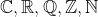sets and operations to generate their number fields.

There exists the set p of prime numbers from the set of integers. Given the square root operation, generate the set of all  for a set called p'. With elements of p'={a,b,c...} restriction conditions:

: the confinement conditions.

The idea here is to create a mathematical description of the physical situation. The math logic is that the square root operation cannot be performed on the set element, imposing non-commutativity on addition here when not considering annihilation--so what would be mathematical additive inverses do not cancel to zero. The description of a hadron (characterized above by capital letters M,P,N) jet (M) or gluon cloud (P,N), with different values for different energy levels (and other n-tuples for exotic forms). I don't know how to treat multiplicative associativity/commutativity here.

A second condition:

: the gap condition.
This creates an identity element, and multiplicative inverses define 0. Additive inverses (or symmetric counter magnitudes) exist, but the operation is barred for the unaltered elements in the interests of creating certain 2-tuples and 3-tuples corresponding to confined hadrons. These confined n-tuples are then incorporated into an extended set.
The set then extends to a group. The group operation consists in generating these groups, though this seems redundant; now +q-q pairs or +u+u+d, +d+d+u can be equated as symmetries under rotation. Normal multiplicative associative rule and left- and right-distributive rules are posited (d/t need for non-commutative multiplication now in force as), applying any p'p'=p un-bars the radicand allowing commutative addition and subtraction (e.g. p2=1, p2+p2=n (n=2,3,...) which can be used to reconstruct the number sets

Let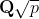be the quadratic fields available, complex and real. Using constructions analogous to references below I would like to construct a vector space with p' elements. In basic terms the elements are analogous to the real vector space, being that p' is intuitively mappable over the real line with magnitude and direction. It may be possible to extend these constructions to a Hilbert space. I do not know how to conceive this as a quadratic form, which may be necessary.

Noting the constructions and developments in references, the construction may be able to move fromto G3 to what should exist (within a subset of)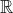4, and then a formulated Hilbert vector space with needed conditions. The aim is what can function as a compact gauge group that encodes the physical situation and can work to generate the gauge symmetry groups of the Standard model, provide for logical gauge transformations and account for renormalization (e.g. see exponentiations as equating to divergences in loop diagrams) or gauge fixing, and account for the mass gap. I do not know enough, I ask for your help, inputs, critique; I have gotten through 2 papers referenced and limited on 2 others.

Ref. 1: A. Jadczyk; On the bundle of Clifford algebras over the space of quadratic forms; Paper presented at the 12th International Conference on Clifford Algebras and their Applications in Mathematical Physics.; incomplete. For each quadratic form Q∈Quad(V) over a given vector space over a field K we have the Clifford algebra Cl(V,Q) defined as the quotient T(V)/I(Q) of the tensor algebra T(V) over the two-sided ideal generated by expressions of the form

Ref. 2: D. Hestenes; Oersted Medal Lecture 2002: Reforming the mathematical language of physics, Am. J. Phys. 71 (2), February 2003, pp. 104--121. ; complete. Geometric Algebra vector space construction, G3, "The rules for multiplying vectors are the basic grammar rules for GA, and they can be applied to vector spaces of any dimension."

Ref. 3: Ibid.; Spacetime Physics with Geometric Algebra, Am. J. Phys. 71 (6), June 2003, pp. 1--24) ; incomplete. extends GA to GR 4-D spacetime; clarifications of Dirac's theory/equation, QM implications.

Ref. 4: Kumar, K.N.P.; Yang–Mills Existence and Mass Gap(Unsolved Problem): Aufklärung La Altagsgeschichte: Enlightenment of a Micro History; International Journal of Scientific and Research Publications, Volume 3, Issue 10, October 2013.; 231pgs.; complete. Dense with formalism and repetitions--apparently Category Theory construction of Yang-Mills problem propositions from CMI or slightly less official source. Again, very repetitive--and I'm not sure this actually is anything-- but it is a categorizing of propositions, each one split three ways (G1,2,3, T1,2,3) the author then looks to transform by logic to lambda (calculus?) addition, reaching the conclusion, "And as one sees, all the coefficients are positive. It follows that all the roots have negative real part, and this proves the theorem." I do not believe the conclusion: this could just be an even worse attempt at proving Y-M existence &  than what I put above. Possibly most value found at Ref. 3: Reifler, F.; Morris, R."Conditions for exact equivalence of Kaluza-Klein and Yang-Mills theories", Lockheed Martin Corporation MS2 137−205. Cited file (Kumar,231pgs.) too large: see https://www.ijsrp.org/research-paper-1013/ijsrp-p2248.pdf

For further research: Yukalov, Yukalova; self-similar approximation theory e.g. From asymptotic series to self-similar approximants.

P.s. I still need the Foundations of Mathematics by Stewart and Tall.

Edited by NTuft
##### Share on other sites

I think you're still confused as concerns 'fields' in algebra vs 'fields' in physics. Very different things.

It's like 'dog' in 'hot dog' vs 'dog' in 'I'm walking my dog.'

##### Share on other sites

To clarify,

I'll say that the square root operation is a non-linear operation and cannot be performed at what I want to be the linear level so it functions as a place-holder, for the quark-antiquark here. I do not mean that -M is an anti-meson, but rather as though it's been rotated 180degrees.

Proton and anti-proton. Analagous to neutron, but the electron needs a work-up. So massive particles here, and there needs to be accounting for mass-energy equivalence. I think particle-antiparticle meeting can bring the annihilation, crossing the zero limit to produce massless emissions.

23 hours ago, joigus said:

I think you're still confused as concerns 'fields' in algebra vs 'fields' in physics. Very different things.

It's like 'dog' in 'hot dog' vs 'dog' in 'I'm walking my dog.'

The mathematical field gives scalars for a vector space. I think the physics field is quantifying x at points in space. We then can build up these vector spaces to the description of physical fields. This is out of order, but you know more about it than I do, Chief; there's a need to get to , and I believe you know about projective spaces to get to more complexity.
I'm looking at how an algebraic number field could build up a description. I think it can be extended to a quadratic form if we take it that the  is the variables of energy-momentum or mass. I don't know about the co-efficients yet or where it goes from linear to non-linear though I think that could help, but I'm not hot-dogging it anymore, I know I don't know enough.
I still hold that this quadratic field may be the real basis for the complex field...

Edited by NTuft
crossing the zero limit to produce massless emissions. +last line...
##### Share on other sites

1 hour ago, NTuft said:

there's a need to get to , and I believe you know about projective spaces to get to more complexity.

I think @studiot knows much more about projective geometry than I do. Also other members who are mathematicians, or much more educated in higher mathematics.

The mass gap in YM is considered to be the toughest problem around concerning physics, BTW.Just saying...

##### Share on other sites

Quote

"We conjecture that in Yang–Mills theories the ratio between the ground-state glueball mass squared and the string tension is proportional to the ratio of the eigenvalues of quadratic Casimir operators in the adjoint and the fundamental representations. The proportionality constant depends on the dimension of the space-time only, and is henceforth universal. We argue that this universality, which is supported by available lattice results, is a direct consequence of area-law confinement. In order to explain this universal behavior, we provide three analytical arguments, based respectively on a Bethe–Salpeter analysis, on the saturation of the scale anomaly by the lightest scalar glueball and on QCD sum rules, commenting on the underlying assumptions that they entail and on their physical implications."

So does area-law confinement imply Polyakov loops to you? As opposed to Wilson loops being based on perimeter? Is the issue that glueballs need to have a prediction made about their lower mass bound?

##### Share on other sites

Sorry I can't comment much on that. I know next to nothing about Polyakov loops. From what I gather, those are Wilson loops applied to a QFT upon which you've performed a Wick rotation. I suppose they give you topological invariants of the corresponding thermal theory?

## Create an account

Register a new account